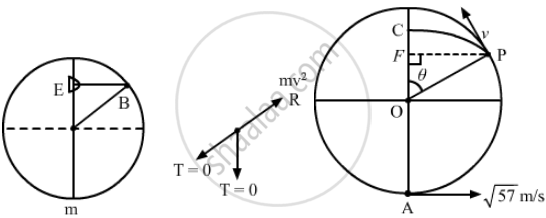Department of Pre-University Education, KarnatakaPUC Karnataka Science Class 11
Advertisement Remove all ads

# A Heavy Particle is Suspended by a 1⋅5 M Long String. It is Given a Horizontal Velocity of √ 57 M/S (A) Find the Angle Made by the String with the Upward Vertical When It Becomes Slack. - Physics

Numerical

A heavy particle is suspended by a 1⋅5 m long string. It is given a horizontal velocity of $\sqrt{57} \text{m/s}$ (a) Find the angle made by the string with the upward vertical when it becomes slack. (b) Find the speed of the particle at this instant. (c) Find the maximum height reached by the particle over the point of suspension. Take g = 10 m/s2

Advertisement Remove all ads

#### Solution

$\text{ Given }:$
$\text{ Length of the string, L = 1 . 5 m}$
$\text{ Initial speed of the particle, u } = \sqrt{57} \text{ m/s }$
$(\text{a}) \text{ mg } \cos \theta = \frac{\text{m}\nu^2}{\text{L}}$
$\nu^2 = \text{Lg} \cos \theta . . . (\text{i})$

Change in K.E. = Work done

$\frac{1}{2}\text{m}\nu^2 - \frac{1}{2}\text{mu}^2 = - \text{mgh}$

$\Rightarrow \nu^2 - 57 = - 2 \times 1 . 5 \text{g} \left( 1 + \cos \theta \right)$

$\Rightarrow \nu^2 = 57 - 3\text{g }\left( 1 + \cos \theta \right) . . . (\text{ii})$Putting the value of $\nu$  from equation (i),

$15 \cos \theta = 57 - 3g \left( 1 + \cos \theta \right)$
$\Rightarrow 15 \cos \theta = 57 - 30 - 30 \cos \theta$
$\Rightarrow 45 \theta = 27$
$\Rightarrow \cos \theta = \frac{3}{5}$
$\Rightarrow \theta = \cos^{- 1} \frac{3}{5} = 53^\circ$

(b) From equation (ii),

$\nu = \sqrt{57 - 3g \left( 1 + \cos \theta \right)}$
$= \sqrt{9} = 3 \text{ m/s}$

(c) As the string becomes slack at point P, the particle will start executing a projectile motion.
$\text{ h = OF + FC}$
$= 1 . 5 \cos \theta + \frac{\text{u}^2 \sin^2 \theta}{2 \text{g}}$
$= \left( 1 . 5 \right) \times \frac{3}{5} + \frac{9 \times \left( 0 . 8 \right)^2}{2 \times 10}$
$= 1 . 2 \text{m}$
Is there an error in this question or solution?
Advertisement Remove all ads

#### APPEARS IN

HC Verma Class 11, 12 Concepts of Physics 1
Chapter 8 Work and Energy
Q 57 | Page 136
Advertisement Remove all ads
Advertisement Remove all ads
Share
Notifications

View all notifications

Forgot password?